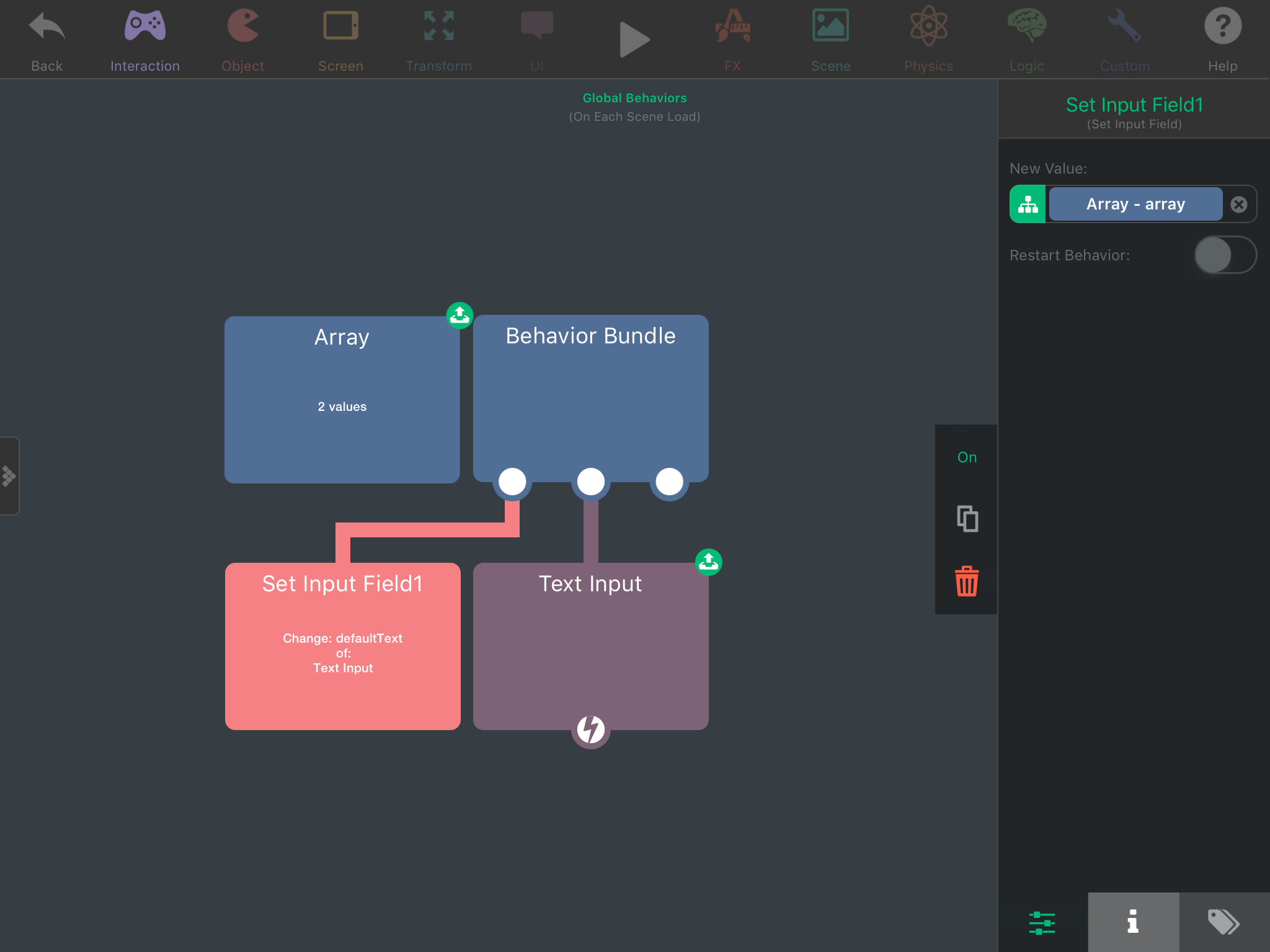Bug: Arrays and dictionaries in set input fields

• Using an array or dictionary in a set input field doesn't work properly. Here's an example:The array is:

0: This is the value at index zero
1: This is the value at index one

The text input gives this:

( "<BehaviourInputField: 0x174a56c50> = { \n\tControlled By = self \n\tValue Key = (null) \n\tValue: This is the value at index zero \n}", "<BehaviourInputField: 0x17444add0> = { \n\tControlled By = self \n\tValue Key = (null) \n\tValue: This is the value at index one \n}" )

Dictionaries do the same thing:

{ "my first key" = "<BehaviourInputField: 0x170e56410> = { \n\tControlled By = self \n\tValue Key = (null) \n\tValue: my first value \n}"; "my second key" = "<BehaviourInputField: 0x170c50440> = { \n\tControlled By = self \n\tValue Key = (null) \n\tValue: my second value \n}"; }

Edit: moved to bug report section, originally posted to help and support.

• This isn't a bug.

If you print an array in, say, C#, this is what you will see; a summary of the printed object.

Now, if you print Array + Array, you will see This is the value at index 0.This is the value at index 1. This is because it now knows to pull and print the contained strings, rather than print the array itself.

Dictionaries should work the same way.

• @Thecheater887 no, arrays and dictionaries are supposed to be returned in json, so it should return:
["This is the value at index zero","This is the value at index one"]

It works fine if I put the array output directly in the text input.

If this wasn't a bug it'd be a huge inconsistency since it returns in json format all other ways except in a set input field.